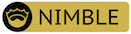### timeit

measuring execution times written in nim.

# timeitmeasuring execution times written in nim.

## Installation

``````nimble install timeit
``````

## Documention

https://xflywind.github.io/timeit/src/main.html

## Usage

### import timeit

In timeit module, you can use the timeGo to measure execution times of proc.
There are three parameters in timeGo.
myFunc is the call of proc. repeatTimes is how many times you want to measure, the result is the average of all the times, loopTimes is how many loops you want to execute in each time. If you don't give the value of loopTimes, the program will calculate the loopTimes according to the execution times in first time.

```template timeGo*(myFunc: untyped,
repeatTimes: int = repeatTimes,
loopTimes: int = loopTimes): Timer```

When you want to pass myFunc parameter to timeGo, there are some points you should take care of.
If you want to pass the proc without return value, you should use the call of the proc, namely myProc(args).
If you want to pass the proc with return value, you should add the pragma {.discardable.} to the definitions of your proc.Then you can use timeGo to measure the execution times of your proc, namely myProc(args).

### another Way

You can also use timeGo as follow:

```import os

echo timeGo do:
os.sleep(12)
var a = 12
for i in 1 .. 1000:
a += 12
# output [12ms 883μs 34.58ns] ± [19μs 974.83ns] per loop (mean ± std. dev. of 7 runs, 10 loops each)```

or you can specify the repeatTimes and loopTimes as follows:

```timeGo(1, 1):
var b = 12
b += 12
# output [200.00ns] ± [0.00ns] per loop (mean ± std. dev. of 1 runs, 1 loops each)```

### use monit function

You can use monit function to measure the times of code executions.
First you can specify the name of this test, for example, "first".Then you can place the start function in the begin of the code you want to measure, and place the finsih function in the end of the code you want to measure.

```import timeit
var m = monit("first")
m.start()
let a = 12
echo a + 3
m.finish()
# first -> [17μs 0.00ns]```

You can also monit once as follows:

```timeOnce("test-once"):
var a = 12
for i in 1 .. 10000:
a += i
echo a```

### use command-line interface

Firstly, you need to make sure that your .nimble directory must be in your path environment. Then you can use timeit --name=yourNimFile --def=yourProc.
However, string parameters can't appear in yourProc.And If you want to specify more than one parameter, you should use "yourProc(parameters)".

```# in bash
timeit --name=test --def=hello()
# [46ms 290μs 893.17ns] ± [1ms 164μs 333.97ns] per loop (mean ± std. dev. of 7 runs, 10 loops each)```

You can also measure the execution time of the whole Nim file.

```# in bash
timeit test.nim
# debug mode
# test-whole -> [208μs 100.00ns]

# or specify the -d flag
timeit test.nim -d:release
# release mode
# test-whole -> [29μs 200.00ns]```

## Examples

for proc without return value

```import timeit

proc mySleep(num: varargs[int]) =
for i in 1 .. 10:
echo timeGo(mySleep(5, 2, 1))
# [216.50ns] ± [1μs 354.44ns] per loop (mean ± std. dev. of 7 runs, 1000000 loops each)```

for proc with return value

```import timeit

proc mySleep(num: varargs[int]): int {.discardable.} =
for i in 1 .. 10: